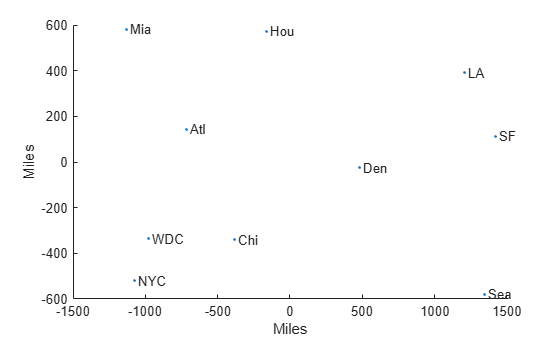# cmdscale

Classical multidimensional scaling

## Syntax

```Y = cmdscale(D) [Y,e] = cmdscale(D) [Y,e] = cmdscale(D,p) ```

## Description

`Y = cmdscale(D)` takes an `n`-by-`n` distance matrix `D`, and returns an `n`-by-`p` configuration matrix `Y`. Rows of `Y` are the coordinates of `n` points in `p`-dimensional space for some `p < n`. When `D` is a Euclidean distance matrix, the distances between those points are given by `D`. `p` is the dimension of the smallest space in which the `n` points whose inter-point distances are given by `D` can be embedded.

`[Y,e] = cmdscale(D)` also returns the eigenvalues of `Y*Y'`. When `D` is Euclidean, the first `p` elements of `e` are positive, the rest zero. If the first `k` elements of `e` are much larger than the remaining `(n-k)`, then you can use the first `k` columns of `Y` as `k`-dimensional points whose inter-point distances approximate `D`. This can provide a useful dimension reduction for visualization, e.g., for `k = 2`.

`D` need not be a Euclidean distance matrix. If it is non-Euclidean or a more general dissimilarity matrix, then some elements of `e` are negative, and `cmdscale` chooses `p` as the number of positive eigenvalues. In this case, the reduction to `p` or fewer dimensions provides a reasonable approximation to `D` only if the negative elements of `e` are small in magnitude.

`[Y,e] = cmdscale(D,p)` also accepts a positive integer `p` between 1 and `n`. `p` specifies the dimensionality of the desired embedding `Y`. If a `p` dimensional embedding is possible, then `Y` will be of size `n`-by-`p` and `e` will be of size `p`-by-1. If only a `q` dimensional embedding with `q < p` is possible, then `Y` will be of size `n`-by-`q` and `e` will be of size `p`-by-1. Specifying `p` may reduce the computational burden when `n` is very large.

You can specify `D` as either a full dissimilarity matrix, or in upper triangle vector form such as is output by `pdist`. A full dissimilarity matrix must be real and symmetric, and have zeros along the diagonal and positive elements everywhere else. A dissimilarity matrix in upper triangle form must have real, positive entries. You can also specify `D` as a full similarity matrix, with ones along the diagonal and all other elements less than one. `cmdscale` transforms a similarity matrix to a dissimilarity matrix in such a way that distances between the points returned in `Y` equal or approximate `sqrt(1-D)`. To use a different transformation, you must transform the similarities prior to calling `cmdscale`.

## Examples

collapse all

This example shows how to construct a map of 10 US cities based on the distances between those cities, using `cmdscale`.

First, create the distance matrix and pass it to `cmdscale`. In this example, `D` is a full distance matrix: it is square and symmetric, has positive entries off the diagonal, and has zeros on the diagonal.

```cities = ... {'Atl','Chi','Den','Hou','LA','Mia','NYC','SF','Sea','WDC'}; D = [ 0 587 1212 701 1936 604 748 2139 2182 543; 587 0 920 940 1745 1188 713 1858 1737 597; 1212 920 0 879 831 1726 1631 949 1021 1494; 701 940 879 0 1374 968 1420 1645 1891 1220; 1936 1745 831 1374 0 2339 2451 347 959 2300; 604 1188 1726 968 2339 0 1092 2594 2734 923; 748 713 1631 1420 2451 1092 0 2571 2408 205; 2139 1858 949 1645 347 2594 2571 0 678 2442; 2182 1737 1021 1891 959 2734 2408 678 0 2329; 543 597 1494 1220 2300 923 205 2442 2329 0]; [Y,eigvals] = cmdscale(D);```

Next, look at the eigenvalues returned by `cmdscale`. Some of these are negative, indicating that the original distances are not Euclidean. This is because of the curvature of the earth.

```format short g [eigvals eigvals/max(abs(eigvals))]```
```ans = 10×2 9.5821 0.0000 1.6868 0.0000 0.0082 0.0000 0.0014 0.0000 0.0005 0.0000 0.0000 0.0000 0.0000 0.0000 -0.0009 -0.0000 -0.0055 -0.0000 -0.0355 -0.0000 ```

However, in this case, the two largest positive eigenvalues are much larger in magnitude than the remaining eigenvalues. So, despite the negative eigenvalues, the first two coordinates of `Y` are sufficient for a reasonable reproduction of `D`.

```Dtriu = D(find(tril(ones(10),-1)))'; maxrelerr = max(abs(Dtriu-pdist(Y(:,1:2))))./max(Dtriu)```
```maxrelerr = 0.0075371 ```

Here is a plot of the reconstructed city locations as a map. The orientation of the reconstruction is arbitrary.

```plot(Y(:,1),Y(:,2),'.') text(Y(:,1)+25,Y(:,2),cities) xlabel('Miles') ylabel('Miles')```Determine how the quality of reconstruction varies when you reduce points to distances using different metrics.

Generate ten points in 4-D space that are close to 3-D space. Take a linear transformation of the points so that their transformed values are close to a 3-D subspace that does not align with the coordinate axes.

```rng default % Set the seed for reproducibility A = [normrnd(0,1,10,3) normrnd(0,0.1,10,1)]; B = randn(4,4); X = A*B;```

Reduce the points in `X` to distances by using the Euclidean metric. Find a configuration `Y` with the inter-point distances.

```D = pdist(X,'euclidean'); Y = cmdscale(D);```

Compare the quality of the reconstructions when using 2, 3, or 4 dimensions. The small `maxerr3` value indicates that the first 3 dimensions provide a good reconstruction.

`maxerr2 = max(abs(pdist(X)-pdist(Y(:,1:2)))) `
```maxerr2 = 0.1631 ```
`maxerr3 = max(abs(pdist(X)-pdist(Y(:,1:3)))) `
```maxerr3 = 0.0187 ```
`maxerr4 = max(abs(pdist(X)-pdist(Y)))`
```maxerr4 = 9.6589e-15 ```

Reduce the points in `X` to distances by using the `'cityblock'` metric. Find a configuration `Y` with the inter-point distances.

```D = pdist(X,'cityblock'); [Y,e] = cmdscale(D);```

Evaluate the quality of the reconstruction. `e` contains at least one negative element of large magnitude, which might account for the poor quality of the reconstruction.

`maxerr = max(abs(pdist(X)-pdist(Y)))`
```maxerr = 9.0488 ```
`min(e)`
```ans = -5.6586 ```

## References

 Seber, G. A. F. Multivariate Observations. Hoboken, NJ: John Wiley & Sons, Inc., 1984.